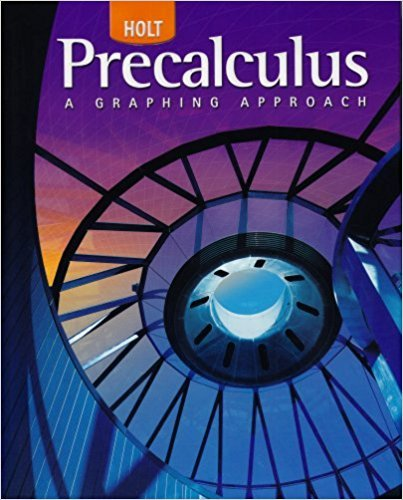×
×

# Solutions for Chapter 3.3: Quadratic Functions## Full solutions for Precalculus | 1st Edition

ISBN: 9780030416477Solutions for Chapter 3.3: Quadratic Functions

Solutions for Chapter 3.3
4 5 0 429 Reviews
18
2
##### ISBN: 9780030416477

Since 54 problems in chapter 3.3: Quadratic Functions have been answered, more than 24579 students have viewed full step-by-step solutions from this chapter. This textbook survival guide was created for the textbook: Precalculus, edition: 1. Chapter 3.3: Quadratic Functions includes 54 full step-by-step solutions. This expansive textbook survival guide covers the following chapters and their solutions. Precalculus was written by and is associated to the ISBN: 9780030416477.

Key Calculus Terms and definitions covered in this textbook
• Compounded annually

See Compounded k times per year.

• Double-blind experiment

A blind experiment in which the researcher gathering data from the subjects is not told which subjects have received which treatment

• Even function

A function whose graph is symmetric about the y-axis for all x in the domain of ƒ.

• Event

A subset of a sample space.

• Gaussian curve

See Normal curve.

• Geometric sequence

A sequence {an}in which an = an-1.r for every positive integer n ? 2. The nonzero number r is called the common ratio.

• Inverse reflection principle

If the graph of a relation is reflected across the line y = x , the graph of the inverse relation results.

• Linear regression equation

Equation of a linear regression line

• Multiplicative inverse of a complex number

The reciprocal of a + bi, or 1 a + bi = a a2 + b2- ba2 + b2 i

• Parameter

See Parametric equations.

• Parametrization

A set of parametric equations for a curve.

• Polynomial interpolation

The process of fitting a polynomial of degree n to (n + 1) points.

• Quotient identities

tan ?= sin ?cos ?and cot ?= cos ? sin ?

• Series

A finite or infinite sum of terms.

• Shrink of factor c

A transformation of a graph obtained by multiplying all the x-coordinates (horizontal shrink) by the constant 1/c or all of the y-coordinates (vertical shrink) by the constant c, 0 < c < 1.

• Terminal point

See Arrow.

• Transverse axis

The line segment whose endpoints are the vertices of a hyperbola.

• Tree diagram

A visualization of the Multiplication Principle of Probability.

• Variable

A letter that represents an unspecified number.

• Zero matrix

A matrix consisting entirely of zeros.

×